# Grade - math word problems

1. Evaluate - order of opsEvaluate the expression: 32+2[5×(24-6)]-48÷24 Pay attention to the order of operation including integers
2. Before yesterdayHe merchant adds a sale sign in his shop window to the showed pair of shoes in the morning: "Today by p% cheaper than yesterday. " After a while, however, he decided that the sign saying: "Today 62.5% cheaper than the day before yesterday". Determine the n
3. Coordinates of square verticesI have coordinates of square vertices A / -3; 1/and B/1; 4 /. Find coordinates of vertices C and D, C 'and D'. Thanks Peter.
4. A rhombusA rhombus has sides of length 10 cm, and the angle between two adjacent sides is 76 degrees. Find the length of the longer diagonal of the rhombus.
5. Positional energyWhat velocity in km/h must a body weighing 60 kg have for its kinetic energy to be the same as its positional energy at the height 50 m?
6. Isosceles triangle 10In an isosceles triangle, the equal sides are 2/3 of the length of the base. Determine the measure of the base angles.
7. The hemisphereThe hemisphere container is filled with water. What is the radius of the container when 10 liters of water pour from it when tilted 30 degrees?
8. Floating of wood - Archimedes lawWhat will be the volume of the floating part of a wooden (balsa) block with a density of 200 kg/m3 and a volume of 0.02 m3 that floats in alcohol? (alcohol density is 789 kg/m3)
9. Cheops pyramid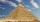The Pyramid of Cheops is a pyramid with a square base with a side of 233 m and a height of 146.6 m. It made from limestone with a density of 2.7 g/cm3. Calculate the amount of stone in tons. How many trains with 30 twenty tons wagons carry the stone?
10. AM of three numbersThe number 2010 can be written as the sum of 3 consecutive natural numbers. Determine the arithmetic mean of these numbers.
11. Frustum of a cone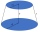A reservoir contains 28.54 m3 of water when completely full. The diameter of the upper base is 3.5 m while at the lower base is 2.5 m. Determine the height if the reservoir is in the form of a frustum of a right circular cone.
12. Common divisorsFind all common divisors of numbers 30 and 45.
13. Numbers divisionWhich number should be divided by 2 3/4 to get 11/12?
14. Cylinder and its circumferenceIf the height of a cylinder is 4 times its circumference. What is the volume of the cylinder in terms of its circumference, c?
15. Propeller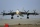The aircraft propeller rotates at an angular speed of 200 rad/s. A) What is the speed at the tip of the propeller if its distance from the axis of rotation is 1.5 m? B) What path does the aircraft travel during one revolution of the propeller at a speed
16. Reducing balance method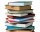A company buys an item having a useful life of 10 years for 1,000,000. If the company depreciates the item by the reducing balance method, a. Determine the depreciation for the first year. b. Estimate the depreciation for the second and third years. c..
17. A baker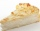A baker has 5 1/4 pies in her shop. She cut the pies in pieces that are each 1/8 of a whole pie. How many pieces of pie does she have?
18. Surface of the cylinderCalculate the surface of the cylinder for which the shell area is Spl = 20 cm2 and the height v = 3.5 cm
19. Cuboid face diagonalsThe lengths of the cuboid edges are in the ratio 1: 2: 3. Will the lengths of its diagonals be the same ratio? The cuboid has dimensions of 5 cm, 10 cm, and 15 cm. Calculate the size of the wall diagonals of this cuboid.
20. Compound interest 3After 8 years, what is the total amount of a compound interest investment of \$25,000 at 3% interest, compounded quarterly? (interest is now dream - in the year 2019)

Do you have an interesting mathematical word problem that you can't solve it? Enter it, and we can try to solve it.

To this e-mail address, we will reply solution; solved examples are also published here. Please enter the e-mail correctly and check whether you don't have a full mailbox.

Please do not submit problems from current active competitions such as Mathematical Olympiad, correspondence seminars etc...